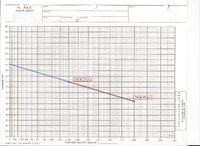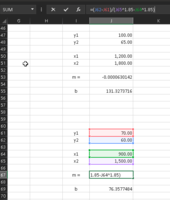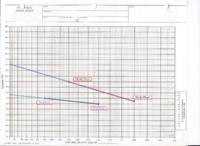# Extrapolate graph line on semi-log graph

#### Cidona

##### New member
Dear Forum.

I hope I have posted this in the correct section; it’s a long time since I took maths and even back then I wouldn’t have covered this. If I have posted this in the incorrect forum area, apologies; please advise where may be suitable.

I have a graph type which is used in my industry (fire protection) for graphing water supplies. It is considered a N1.85 Graph. It is semi-log (10xN1.85). The flow (x-axis) is logarithmic. The scale of distances between the increments increases. The rate of increase is the value to the power of 1.85 less the preceding value. (see table below with column in yellow showing sample of the increase rate in width).

 First increment​ to ^1.85​ less preceding value (width)​ 1​ 1​ 1​ 2​ 3.60500185​ 2.60500185​ 3​ 7.632631955​ 4.027630104​ 4​ 12.99603834​ 5.363406387​ 5​ 19.63787576​ 6.641837414​ 6​ 27.51565232​ 7.877776565​ 7​ 36.59581083​ 9.080158512​ 8​ 46.85074227​ 10.25493144​ 9​ 58.25707056​ 11.40632829​ 10​ 70.79457844​ 12.53750788​ 11​ 84.44549146​ 13.65091302​ 12​ 99.19397753​ 14.74848608​ 13​ 115.0257839​ 15.83180639​ 14​ 131.9279658​ 16.90218184​ 15​ 149.888678​ 17.96071225​

Please see below sample of an actual graph. On the graph I have inserted two points sample points and have a line joining them. The sample points are (a) 100psi (y-axis) @ 1,200 gpm (x-axis) and (b) 65psi (y-axis) @ 1,800 gpm (x-axis).

I’m trying to find a formula that will tell me where this line would intercept on the y axis with 0 pressure. i.e. how much pressure would there be at no flow.In the sample graph above I have drawn a line in red between the two know points and have drawn a light blue line to visually extrapolate as I'm looking for a formula to solve. In this sample the answer would be approx 132 (PSI) (at 0 flow).

Any help in this regard would be greatly appreciated!

#### Dr.Peterson

##### Elite Member
Thanks for providing all the information. I was able to search for information about "N1.85" and confirm your explanation of the graph. One page that discusses it is https://www.dplot.com/n185-hydraulic-scale.htm.

It isn't semi-log at all, though I can see why it looked similar to that to you; it's a power scale, where the actual horizontal distance plotted is proportional to x^1.85. Thus, a straight line that looks like y = mx + b really represents the equation y = mx^1.85 + b.

Your line passes through the points $$\displaystyle (1200^{1.85}, 100)$$ and $$\displaystyle (1800^{1.85}, 65)$$. The slope m is $$\displaystyle \frac{y_2 - y_1}{x_2 - x_1} = \frac{65 - 100}{1800^{1.85} - 1200^{1.85}} = \frac{-35}{555430} = -0.0000630142$$.

Then the y-intercept, which is what you are looking for, is $$\displaystyle b = y - mx^{1.85} = 100 + 0.0000630142 \cdot1200^{1.85} = 131.33$$. Yes, that is very close to your 132.

[EDIT: corrected slope]

Last edited:
•Denis

#### Cidona

##### New member
That is GENIUS! Thank you so, so much Dr. Peterson!!

I will be able to do a nice spreadsheet based on that.

That is interesting information about it not technically being semi-log as I've often heard it referred to as such in the industry and it is always nice to be correctly informed about topics I'd be discussing. Here is a sample of a paper referring to it as semi-log (see page 33).
Zurich Water Supply Testing

I did a second hypothetical line and sure enough your formulas work a treat (see below screen shot). In this case b=76.3577484, which is where it is landing on the graph.There was just a couple of things which I think may have been typos (or my incorrect interpretation), that I'm only mentioning in case someone else stumbles upon this thread after googling to do the same.
(i) I believe where you have -35/555430=-630,142, that it should be -0.0000630142
(ii) I believe where you state "100+630,142*1200^1.85", that it should instead be 100 minus ....(instead of plus), however since you had provided the formula "b=y-mx^1.85, I was able to see the minus sign and use that.

Again, thank you very much!!!

BTW, for my better knowledge - what branch of mathematics would this type of solving be considered?

#### Dr.Peterson

##### Elite Member
Yes, I misread my calculator's result, which had 6.3014241218515384476891777541724e-5, taking the well-hidden exponent as +5; but then I used the number in the calculator as I continued the calculation, so the error didn't affect my result. I've fixed it above.

The sign I used is correct: I subtracted a negative, which I wrote as adding the positive. Both the formula and the work are correct. (Note that the intercept is above the given points, hence the addition.)

This work is called "basic algebra"! Most of it is the slope-intercept form of the equation of a line, but the exponents hide its simplicity, and required some careful thinking about what things mean.

It's funny -- in the old days, graphing was the way to make work easier -- draw the line and get the (approximate) answer. Now, it can just hide formulas that would make it easier to use software to solve a problem, more exactly. In searching for information on this topic, I ran across people asking how to make these graphs on Excel, and the like.

It's also funny how very technical terms like "semi-log" can turn into qualitative descriptions, losing their real meaning entirely. The author of your reference is evidently using the term to mean merely "non-linear along one axis".

#### Cidona

##### New member
Thank for your further response Dr. Peterson!

Basic algebra, eh. I should have at least know the name.

Now that you've explained it, the reason for the the plus sign makes sense. Thank you.

Yes, graphing it by hand was for a long time the norm in the fire protection industry. However being able to put a formula to it and have Excel graph and do multiple different things with that information with the simple change of a cell in Excel, means it's no longer easier (when all parts are considered).

Thank you again for your assistance and informative responses. It's been an education!

Best Regards.